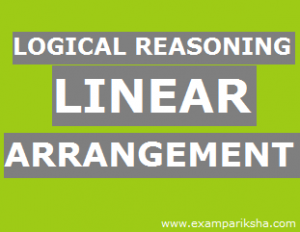Linear Arrangement is essentially ordering the items given in a sequence (in a single line). The questions of this type are also referred to as “Seating Arrangement”. The word “seating arrangement” should not be misconstrued – it should not be treated as consisting of questions involving only persons sitting as per specified conditions.

Essentially, these questions involve arranging subjects or people or things while satisfying the given conditions. The arrangement is done only on one “axis” and hence, the position of the subjects assumes importance here in terms of other like first position, second position, etc.

Let us look at some examples to understand the concept.

Seven persons Paul, Queen, Sam, Tom, Rax, Unif, and Vali are sitting in a row facing us. Rax and Sam sit next to each other. There must be exactly four persons between Queen and Vali. Sam sits in the immediate right of Queen.

Q1. If Paul and Tom are separated exactly by two persons, then who sits to the immediate left of Vali?

A1. Let us write down the conditions given in short form and then represent them pictorally. Also, let us treat the left of the persons sitting as “left” and their “right” for interpreting the conditions.

Rax and Sam sit next to each other, which menas its either [R-S] or [S-R]

There are exactly 4 persons between Queen and Vali, which means [ Q—-V] or [V—-Q]

Sam sits to the immediate right of Queen , which means [SQ]

Now let us analyse the data and conditions given and then put the three conditions together. Let us number the seats from our left to the right as Seat 1 to 7. Since S is to the right of Q and since R and S have to be next to each other, R can come only to the immediate right of S.

Thus, R, S and Q will be in the order RSQ. Since there are four persons between Q and V, Q can be placed in seats 1,2,6 or 7. But if Q is in seat 1 or 2 , then there are no seats for R and S. Hence, there are only two seats available for Q. Let us now fix the positions of R, S and V in each of these two positions of Q and write them down.

The directions Left and Right are as shown below.

Arrangement I:

 1 2 3 4 5 6 7 V R S Q

Arrangement II:

 1 2 3 4 5 6 7 V R S Q

These are the only two arrangements possible for the four persons V, R, S and Q. The other three persons Paul, Tom, and Unif can sit in the three vacant seats in any order, as no information is given about them.Now let us look at the question, Paul and Tom are separated by exactly two persons. Arrangement I is the only one possible as in Arrangement II, Paul and Tom cannot have exactly two persons between them. So, we have the arrangement as follows:

T/P – V – U – P/T – R – S – Q

So, Unif must be sitting to the immediate left of Vali. The answer is Unif.